Examples

Chapter 6 Class 12 Application of Derivatives
Serial order wise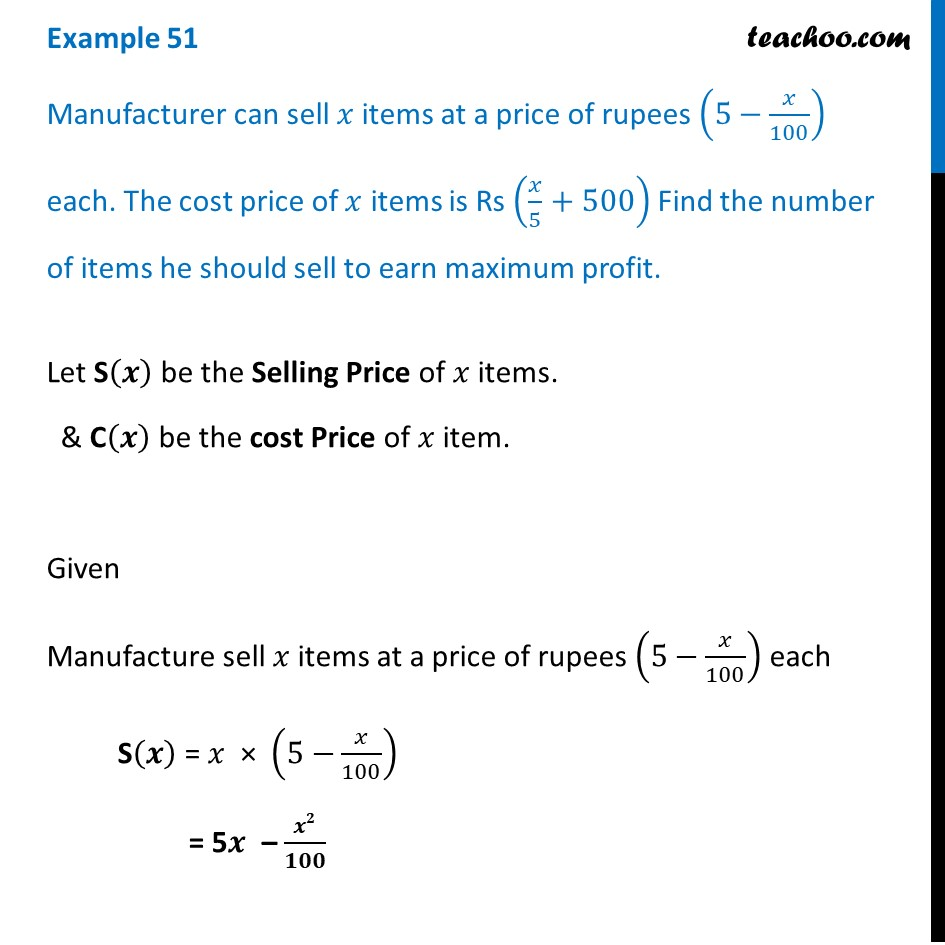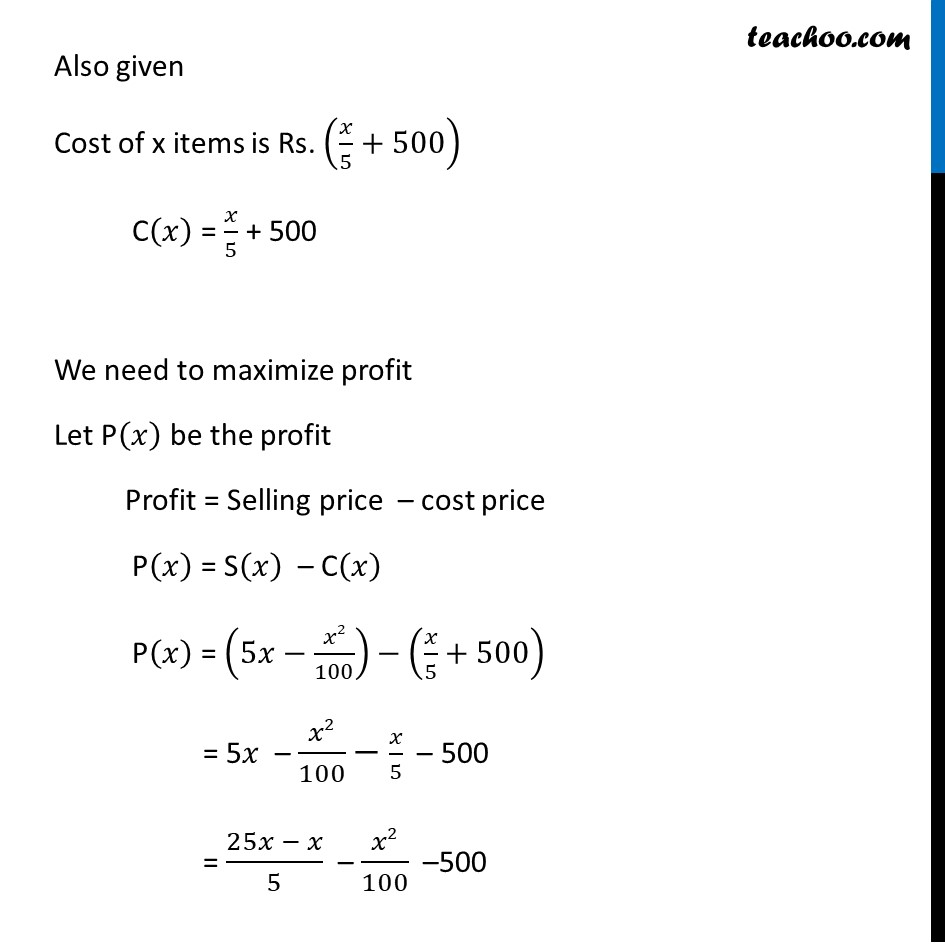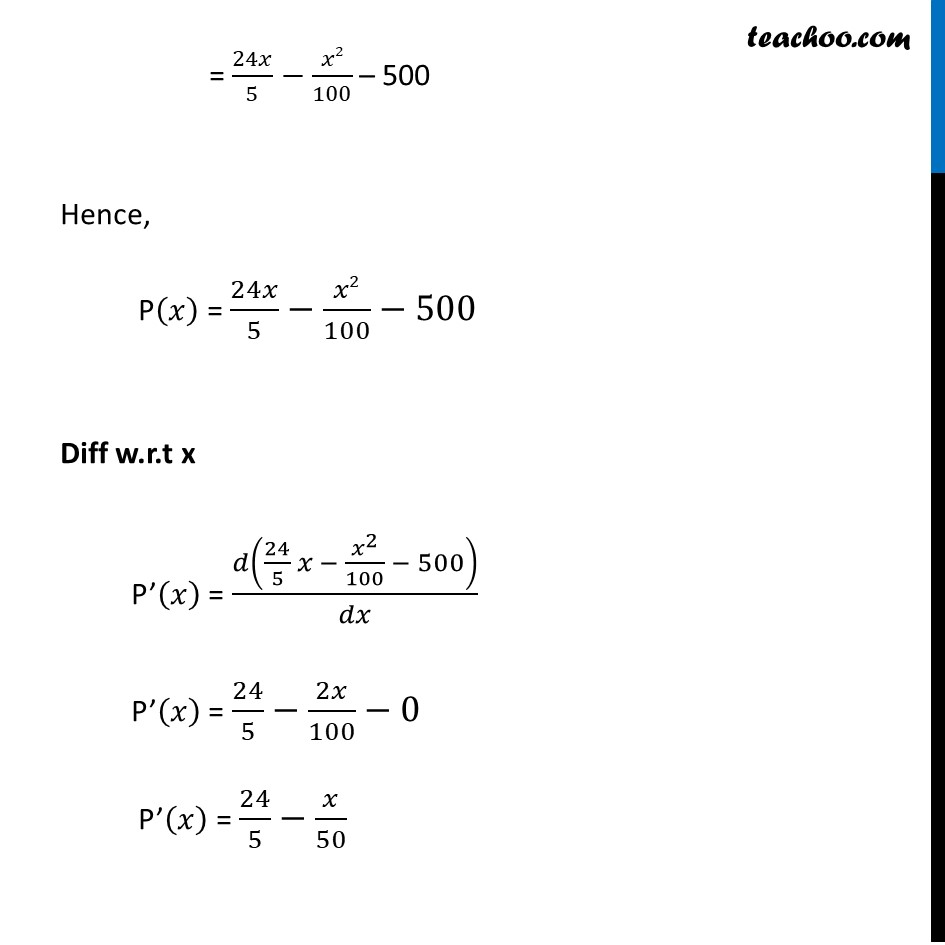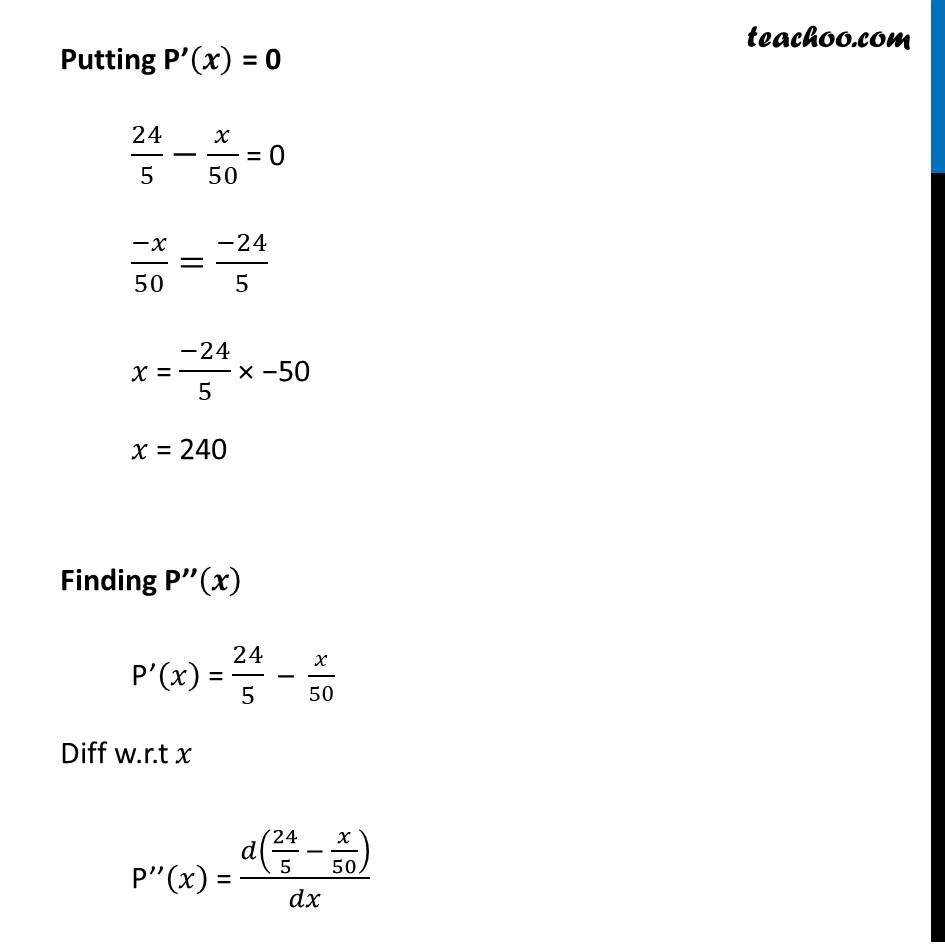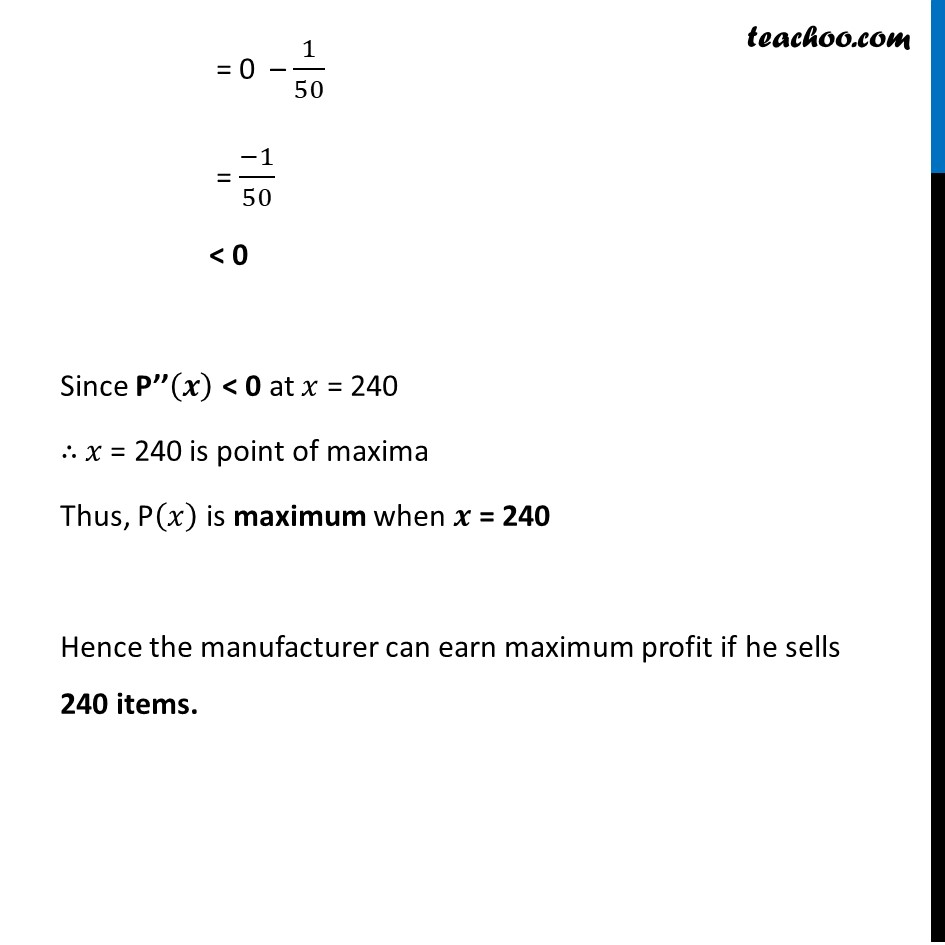Learn in your speed, with individual attention - Teachoo Maths 1-on-1 Class

### Transcript

Example 37 Manufacturer can sell 𝑥 items at a price of rupees (5−𝑥/100) each. The cost price of 𝑥 items is Rs (𝑥/5+500) Find the number of items he should sell to earn maximum profit.Let S(𝒙) be the Selling Price of 𝑥 items. & C(𝒙) be the cost Price of 𝑥 item. Given Manufacture sell 𝑥 items at a price of rupees (5−𝑥/100) each S(𝒙) = 𝑥 × (5−𝑥/100) = 5𝒙 – 𝒙𝟐/𝟏𝟎𝟎 Also given Cost of x items is Rs. (𝑥/5+500) C(𝑥) = 𝑥/5 + 500 We need to maximize profit Let P(𝑥) be the profit Profit = Selling price – cost price P(𝑥) = S(𝑥) – C(𝑥) P(𝑥) = (5𝑥−𝑥2/100)−(𝑥/5+500) = 5𝑥 – 𝑥2/100− 𝑥/5 – 500 = (25𝑥 − 𝑥)/5 – 𝑥2/100 –500 = 24𝑥/5−𝑥2/100 "– 500" Hence, P(𝑥) = 24𝑥/5−𝑥2/100−500 Diff w.r.t x P’(𝑥) = 𝑑(24/5 𝑥 − 𝑥^2/100 − 500)/𝑑𝑥 P’(𝑥) = 24/5−2𝑥/100−0 P’(𝑥) = 24/5−𝑥/50 Putting P’(𝑥) = 0 24/5−𝑥/50 = 0Putting P’(𝒙) = 0 24/5−𝑥/50 = 0 (−𝑥)/50=(−24)/5 𝑥 = (−24)/5 × −50 𝑥 = 240 Finding P’’(𝒙) P’(𝑥) = 24/5 – 𝑥/50 Diff w.r.t 𝑥 P’’(𝑥) = 𝑑(24/5 − 𝑥/50)/𝑑𝑥 = 0 – 1/50 = (−1)/50 < 0 Since P’’(𝒙) < 0 at 𝑥 = 240 ∴ 𝑥 = 240 is point of maxima Thus, P(𝑥) is maximum when 𝒙 = 240 Hence the manufacturer can earn maximum profit if he sells 240 items.